# High School Math : Linear Functions

## Example Questions

### Example Question #1 : Understanding Vertical And Horizontal Lines

Which of the following is a horizontal line?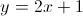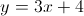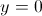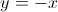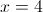Explanation:

A horizontal line has infinitely many values for, but only one possible value for. Thus, it is always of the form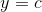, whereis a constant. Horizontal lines have a slope of. The only equation of this form is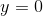### Example Question #2 : Understanding Vertical And Horizontal Lines

Which of the following is a vertical line?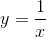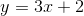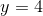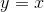Explanation:

A vertical line is one in which thevalues can vary. Namely, there is only one possible value for, andcan be any number. Thus, by this description, the only vertical line listed is### Example Question #3 : Understanding Vertical And Horizontal Lines

Which of the following has a slope of 0?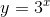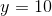Explanation:

A line with a slope of zero will be horizontal. A horizontal line has only one possible value for, andcan be any value.

Thus, the only given equation which fits this description is.

### Example Question #4 : Understanding Vertical And Horizontal Lines

Which of the following is a vertical line?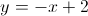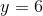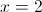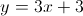Explanation:

A vertical line has infinitely many values ofbut only one value of. Thus, vertical lines are of the form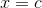, whereis a real number. The only equation of this form is### Example Question #1 : Graphing Linear Functions

Solve for the- and- intercepts: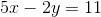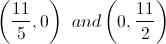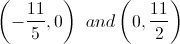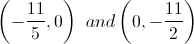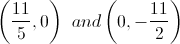Explanation:

To solve for the-intercept, setto zero and solve for: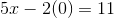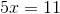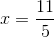To solve for the-intercept, setto zero and solve for: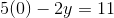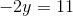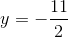### Example Question #2 : Graphing Linear Functions

Solve: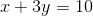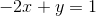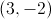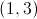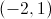Infinitely many solutions

No solutionsExplanation:

Use substution to solve this problem:becomes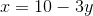and then is substituted into the second equation. Then solve for: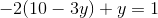, so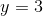andto give the solution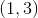.

### Example Question #1 : Transformations Of Linear Functions

Write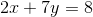in slope-intercept form.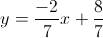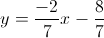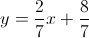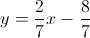Explanation:

Slope-intercept form is.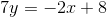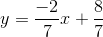### All High School Math Resources